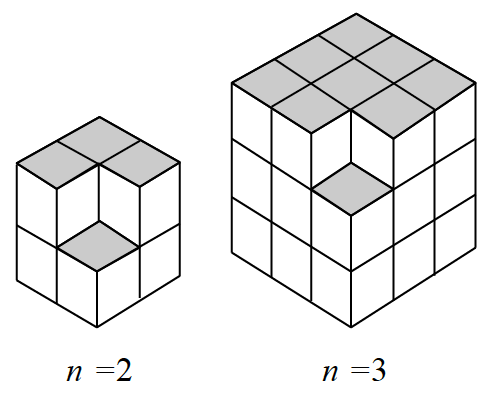### Home > CCA2 > Chapter Ch4 > Lesson 4.1.1 > Problem4-12

4-12.

Examine the figures at right, and then visualize the figure for $n=4$.

1. How many cubes are in the figure for $n = 4$?

Assume that cubes which are blocked from view but would complete the figure are present.

The $n = 2$ figure is a $2 \times 2 \times 2$ cube with one corner cube missing. What will the $n = 4$ figure look like?

2. How many cubes are in the figure for $n = 1$?

Pay close attention to the position of the missing cube. Where would the missing cube be in the $n = 1$ figure?

3. Find the general equation for the number of cubes for any $n$. Verify your formula with the cases of $n = 1$ and $n = 5$.

Imagine that the smaller cubes have side length $1$. What is the volume of each large cube?

4. Is the sequence arithmetic, geometric, or neither? Explain your reasoning.

Do you need to add or multiply to determine the number of cubes in the 'next' figure?